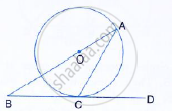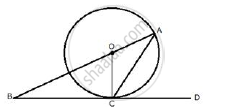Share

# The Given Figure Shows a Circle with Centre O and Bcd is Tangent to It at C. Show That: ∠Acd + ∠Bac = 90° - Mathematics

Course

#### Question

The given figure shows a circle with centre O and BCD is tangent to it at C. Show that: ∠ACD + ∠BAC = 90°#### SolutionJoin OC.
BCD is the tangent and OC is the radius.

∴ OC ⊥ BD

⇒ ∠OCD = 90°

⇒ ∠OCA + ∠ACD = 90°

But in ΔOCA
OA = OC (radii of same circle)

∴ ∠ OAC + ∠ OAC

Substituting (i)

∠ OAC + ∠ACD = 90°

⇒ ∠BAC + ∠ACD = 90°

Is there an error in this question or solution?